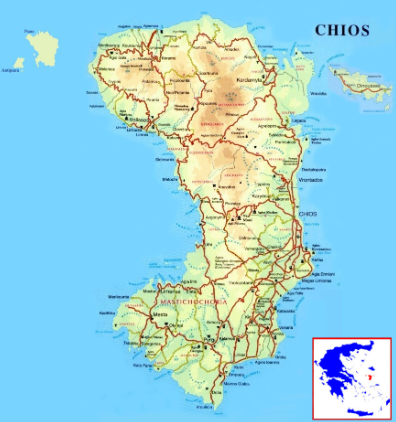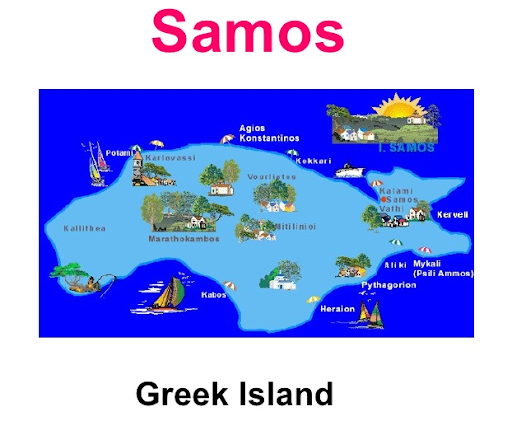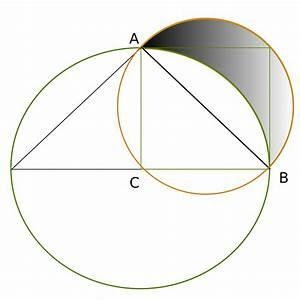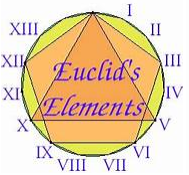Math & Beyond

# Hippocrates of Chios

467 views

 1 Introduction 2 Life of Hippocrates 3 His contribution and achievements 4 Achievements in mathematics 5 Astronomy 6 Summary 7 External References

16 December 2020

## Introduction

Hippocrates of Chios was an ancient Greek mathematician, geometer, and astronomer. He was born on the isle of Chios, where he was originally a merchant.

After some misadventures (he was robbed by either pirates or fraudulent customs officials) he went to Athens, possibly for litigation, where he became a leading mathematician.

There was also a famous doctor by the same name. The documentation of Hippocrates’ life is not concrete, and there may be some inaccurate and incomplete information.

However, his contributions to mathematics and astronomy are note-worthy.Source: Blogspot

## Life Of Hippocrates

He was born in 470 B.C. at Chios, in Greece. He was teaching mathematics in Athens during the period from 450 B.C to 430 B.C. His native place is Chios in Greece near the island Samos which was influenced by Pythagorean thought.

Hippocrates might have been a student of mathematician and astronomer Oenopides. These factors contribute to the Pythagorean traces in his mathematical contributions.It is said that he was

1. A merchant and wealthy in his early days.
2. Robbed of his wealth by pirates in the sea.
3. Fraudulent customs officials looted his wealth.

He then went to Athens for litigation and taught mathematics there for his livelihood from 450 BC to 430 BC. He died in 420 BC.

## Contributions and Achievements

He was initially a merchant then a teacher in mathematics, and he was an astronomer also.

### Achievements in Mathematics

There are three distinct theories of mathematics contributed by Hippocrates as below:

1. Lune of Hippocrates
2. Problem of duplication of the cube (Squaring of the cube) and
3. Reduction theory (technique of reduction).

### 1)Lune of Hippocrates

It is a partial solution of the ‘squaring of circle’ task, as put forth by Hippocrates. He proved that the area of the shaded portion i.e., lune = the area of the triangle ABC.Source: wikipedia

He wrote the first textbook in geometry, named as ‘Elements’. It is a systematically organized writing on basics for building mathematical blocks. It helped for standardized scientific progress in mathematics.

Eudemus of Rhodes, a student of Aristotle wrote History of Geometry. In it he explains about the discovery of Lunes by Hippocrates. Simplicius of Cilicia was a 5th century neo Platonist philosopher.

In his work, a portion of Hippocrates’ Elements is explained by repeating Eudemus’s description about Hippocrates lunes, word for word, and additions from Euclid’s Elements to clearly explain it.

It is a research program to achieve ‘the quadrature of the circle’ i.e, to calculate a circle’s area by constructing a square with equal area. The method involves dividing the circle into several crescent-shaped parts (Lunes) and calculating the areas of all crescents which gives the area of the whole circle.

This theorem states that the ratio of areas of two circles is equal to the ratio of the square of their radii. This method was found not correct by mathematician Ferdinand von Lindamann in 1829.

A century after Hippocrates, 4 other mathematicians improved the Hippocrates’ Elements book in their works.

His Elements book was the basis for Euclid’s Elements in 325 BC, which was a standard geometry book for an extended period. The credit for introducing letters to mark the geometric points and figures in propositions goes to Hippocrates. E.g. vertices of the triangle were denoted as A, B, C by Hippocrates.

Hippocrates’s book gave geometrical solutions to quadratic equations and methods of integration.

Proclus, the last famous Greek philosopher, had also confirmed that

1. Hippocrates of Chios has discovered the quadratrature of the lune, and
2. He actually compiled the “Elements” book.Source: qphs.fs.quoracdn.net

### 2)  Problem of duplication of the cube (Squaring of the cube)

It is for constructing a cube root, by determining two mains proportional between a number and its double. It influenced the attempts to duplicate cubes and proportional problems.
Doubling the cube is by finding the cube root to 2, starting with the unit length of cube of unit volume. The problem involves obtaining an edge of a cube of volume 2 which is the line segment of length  ∛2.Source: cetking

Hippocrates found a step for doubling the cube. That is, he got two mean proposals x and y. They are evenly spaced geometrically between the original side length a, and its double 2a such that:

\begin{align}\frac{a}{x} = \frac{x}{y} = \frac{y}{{2a}}\end{align}

He generalized this concept, though unaware of numbers then, later Elucid has proved there is one mean proposal between two square numbers and two between two cube numbers.

Thus, doubling the cube reduces a three-dimensional problem of doubling the cube to a one-dimensional problem of finding two lengths.

### 3)  Reduction theory (technique of reduction)

It helps to transform specific mathematical questions into more general problems to solve them efficiently.

Hippocrates was the first mathematician to use the technique of reduction in geometric problems. This has been confirmed by 5th-century philosopher Proclus hycaeus. It is described as a transition from one problem or theorem to another of known or solved.

The technique of reduction or proof by contradiction is a related concept. It is still in use among mathematicians. For example, this can be used to prove that there is no smallest rational number.

 Example

Let p and q be two integers, \begin{align}\frac{{{p^2}}}{{{q^2}}} = 2,\rm{then}\,{p^2} = 2{q^2} = \end{align} even number p is an even number.

As squares of odd integers are not even.

Therefore, p = 2k, k being some other integer.

P2 = 2q2 = (2k)2

q2 = 2k2

Hence q is even.

p & q have common factor of 2 here and  \begin{align}\frac{p}{q}\end{align} is not an irreducible fraction.

## Astronomy

It is speculated that Hippocrates studied astronomy during his life in Chios. Hippocrates had tried to explain the phenomenon of comets and the Milky Way.

Thought previously by astronomers as a single body sun from the earth, a planet of long and irregular orbit, and at a low elevation above the horizon, like planet mercury, which cannot be seen. It is seen when the sun is risen, only when they are low before sunrise or after sunset.

Aristotle confirmed Hippocrates' theory on comets as a single body, and this comet was an illusion caused by moisture.

He thought that they were optical illusions. He explained that they were due to refraction of solar rays by moisture inhaled by a putative planet near the Sun and the Stars. His teacher Oenopides had studied both geometry and astronomy in Egypt.

## Summary

Hippocrates was a Greek mathematician, who gave the theories on problems of squaring the circle and duplicating the cube and technique of reduction. He had also worked in Astronomy and in teaching mathematics.

He was the first to write a book on Geometry. His book formed the basis for development of mathematics after his time.

Cuemath, a student-friendly mathematics platform, conducts regular Online Live Classes for academics and skill-development and their Mental Math App, on both iOS and Android, is a one-stop solution for kids to develop multiple skills.

Know more about the Cuemath fee here, Cuemath Fee

## External References

Written by P. Reeta Percy Malathi, Cuemath Teacher

Related Articles
Award-winning math curriculum, FREE for a year